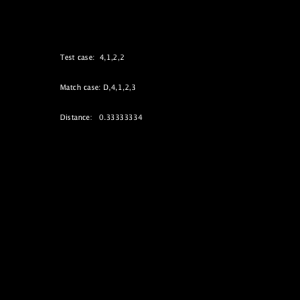Neural network style transfer in OpenCV with Processing

The example is the Processing implementation of the OpenCV sample, fast_neural_style.py to work with live style transfer using existing pre-trained Torch models.

The complete source code is in my GitHub repository of this website at ml20180827a.

Searching in Weka with Processing

Further to the last Weka example, I used the same CSV data file for neighbourhood search. By pressing the mouse button, it generated a random sequence of numbers between 1 to 4. The program used the sequence as an instance to match against the database from the CSV data file. The closet match will be shown together with the distance between the test case (random) and the closet match from the database.

A sample screenshotSource codes

```import weka.core.converters.CSVLoader; import weka.core.Instances; import weka.core.DenseInstance; import weka.core.Instance; import weka.core.neighboursearch.LinearNNSearch; import java.util.Enumeration; import java.io.File;   Instances data; String csv; LinearNNSearch lnn; boolean search; int idx; float dist; String testCase; String matchCase; String distance;   void setup() { size(500, 500); csv = "Testing.csv"; try { loadData(); buildModel(); } catch (Exception e) { e.printStackTrace(); } search = false; idx = -1; dist = 0.0; testCase = ""; matchCase = ""; distance = ""; fill(255); }   void draw() { background(0); if (search) { text(testCase, 100, 100); text(matchCase, 100, 150); text(distance, 100, 200); } }   void loadData() throws Exception { // load external CSV data file, without header row. CSVLoader loader = new CSVLoader(); loader.setNoHeaderRowPresent(true); loader.setSource(new File(dataPath(csv))); data = loader.getDataSet(); data.setClassIndex(0);   println("Attributes : " + data.numAttributes()); println("Instances : " + data.numInstances()); println("Name : " + data.classAttribute().toString());   Enumeration all = data.enumerateInstances(); while (all.hasMoreElements()) { Instance single = (Instance) all.nextElement(); println("Instance : " + (int) single.classValue() + ": " + single.toString()); } }   void buildModel() throws Exception { // Build linear search model. lnn = new LinearNNSearch(data); println("Model built ..."); }   void test() throws Exception { // Construct a test case and do a linear searching. double [] val = new double[data.numAttributes()]; val = 0; testCase = "Test case: "; matchCase = "Match case: "; distance = "Distance: "; for (int i=1; i<val.length; i++) { val[i] = floor(random(4))+1; testCase += (nf((float)val[i]) + ","); } testCase = testCase.substring(0, testCase.length()-1); DenseInstance x = new DenseInstance(1.0, val); x.setDataset(data); Instance c = lnn.nearestNeighbour(x); double [] tmp = lnn.getDistances(); dist = (float) tmp; idx = (int) c.classValue(); matchCase += data.instance(idx).toString(); distance += nf(dist); saveFrame("weka####.png"); }   void mousePressed() { try { test(); } catch (Exception e) { e.printStackTrace(); } search = true; }```

First trial of Weka in Processing

Instead of using the machine learning module (ML) of OpenCV, I also investigated another popular machine learning library for Java, Weka, from the University of Waikato. The first trial was to load an external CSV file into the proper data structure of the Weka library. The content of the CSV file is as follows. The first column will be the index of the records.

```A,1,2,3,4
B,2,3,4,1
C,3,4,1,2
D,4,1,2,3
E,4,3,2,1
```

The first thing to do was to download the latest Weka distribution, currently 3.8 and placed the weka.jar file into the code folder of the Processing sketch.

The complete codes

```import weka.core.converters.CSVLoader; import weka.core.Instances; import weka.core.Instance; import java.util.Enumeration; import java.io.File;   Instances data; // Name of the CSV data file String csv;   void setup() { size(600, 600); csv = "Testing.csv"; try { loadData(); } catch (Exception e) { e.printStackTrace(); } noLoop(); }   void draw() { background(0); }   void loadData() throws Exception { CSVLoader loader = new CSVLoader(); loader.setNoHeaderRowPresent(true); loader.setSource(new File(dataPath(csv))); data = loader.getDataSet(); data.setClassIndex(0);   println("Attributes : " + data.numAttributes()); println("Instances : " + data.numInstances()); println("Name : " + data.classAttribute().toString()); // To scan through all the records of the CSV file Enumeration all = data.enumerateInstances(); while (all.hasMoreElements()) { Instance rec = (Instance) all.nextElement(); println("Instance : " + rec.classValue() + ": " + rec.toString()); } }```

The console output

```Attributes : 5
Instances : 5
Name : @attribute att1 {A,B,C,D,E}
Instance : 0.0: A,1,2,3,4
Instance : 1.0: B,2,3,4,1
Instance : 2.0: C,3,4,1,2
Instance : 3.0: D,4,1,2,3
Instance : 4.0: E,4,3,2,1
```